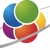# Area of a circleUsing circumference to find a circle's area. Know the formulas for the area and circumference of a circle and use them to solve problems; give an informal derivation of the relationship between the circumference and area... Using circumference to find a circle's area. Know the formulas for the area and circumference of a circle and use them to solve problems; give an informal derivation of the relationship between the circumference and area of a circle.
More... Collapse
0 Views